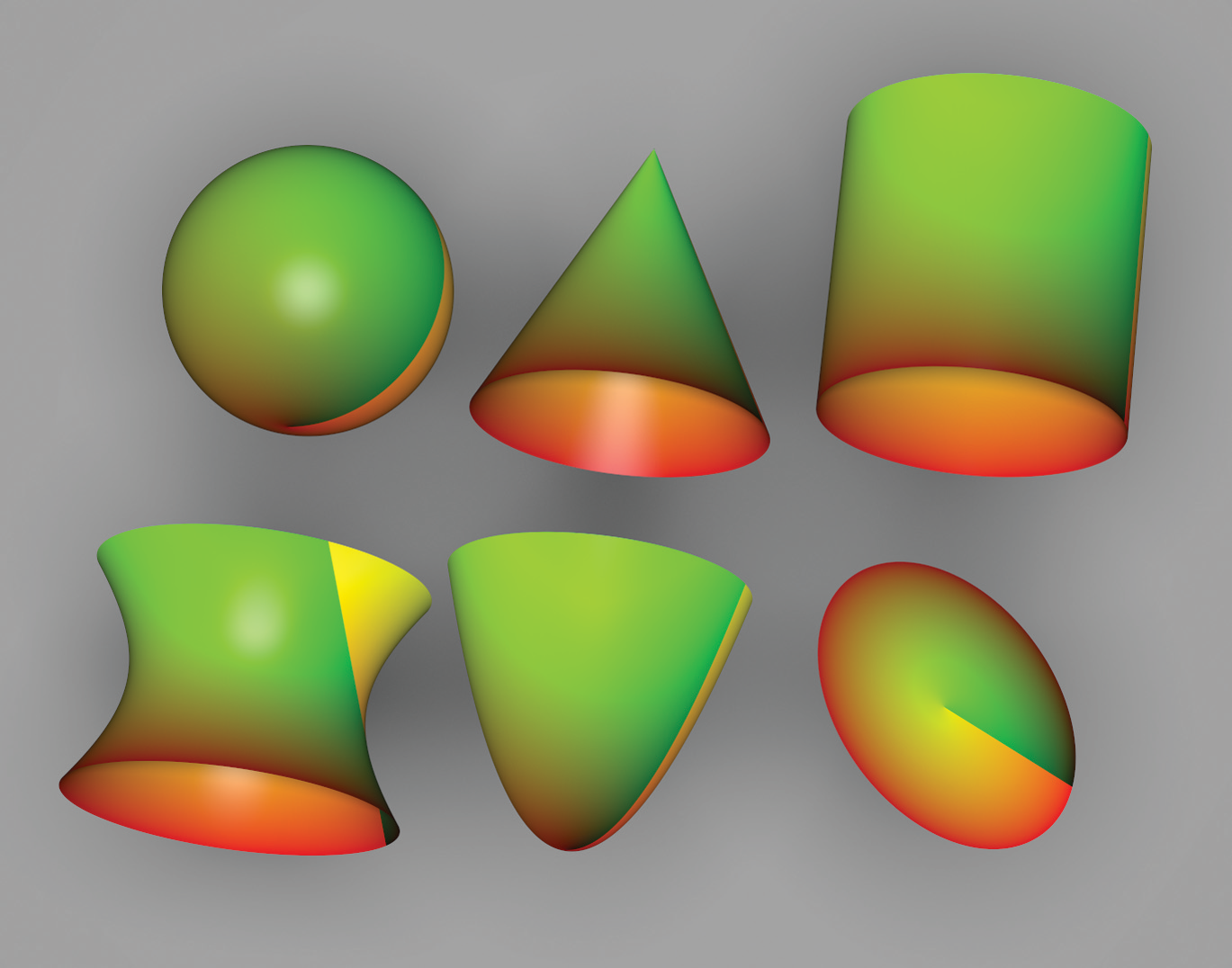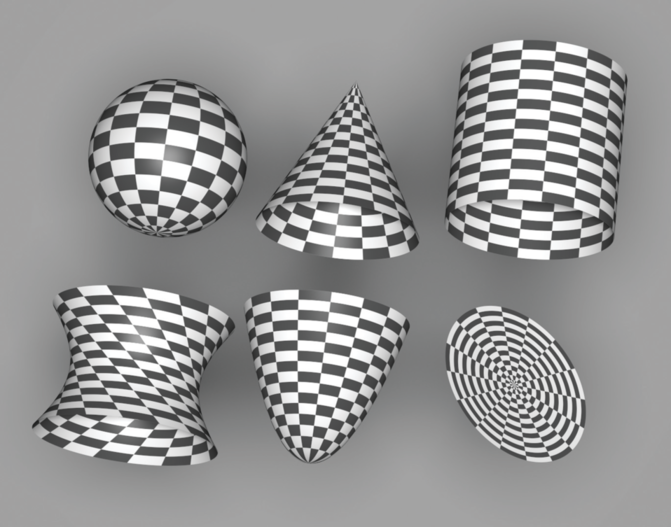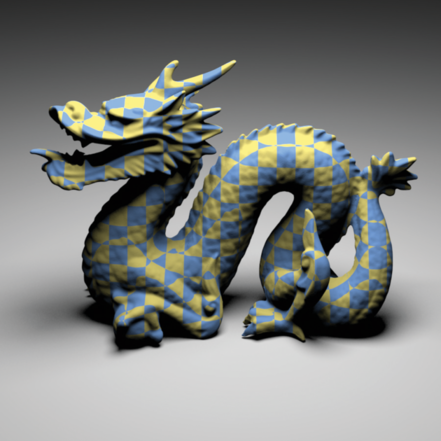## 10.5 Solid and Procedural Texturing

Once one starts to think of the texture coordinates used by 2D texture functions as quantities that can be computed by arbitrary functions and not just from the parametric coordinates of the surface, it is natural to generalize texture functions to be defined over 3D domains (often called solid textures) rather than just 2D . One reason solid textures are particularly convenient is that all objects have a natural 3D texture mapping—object space position. This is a substantial advantage for texturing objects that don’t have a natural 2D parameterization (e.g., triangle meshes and implicit surfaces) and for objects that have a distorted parameterization (e.g., near the poles of a sphere). In preparation for this idea, Section 10.2.5 defined a general TextureMapping3D interface to compute 3D texture coordinates as well as a TransformMapping3D implementation.

Solid textures introduce a new problem, however: texture representation. A 3D image map takes up a fair amount of storage space and is much harder to acquire than a 2D texture map, which can be extracted from photographs or painted by an artist. Therefore, procedural texturing—the idea that programs could be executed to generate texture values at arbitrary positions on surfaces in the scene—came into use at the same time that solid texturing was developed. A simple example of procedural texturing is a procedural sine wave. If we wanted to use a sine wave for bump mapping (for example, to simulate waves in water), it would be inefficient and potentially inaccurate to precompute values of the function at a grid of points and then store them in an image map. Instead, it makes much more sense to evaluate the sin() function at points on the surface as needed.

If we can find a 3D function that describes the colors of the grain in a solid block of wood, for instance, then we can generate images of complex objects that appear to be carved from wood. Over the years, procedural texturing has grown in application considerably as techniques have been developed to describe more and more complex surfaces procedurally.

Procedural texturing has a number of interesting implications. First, it can be used to reduce memory requirements for rendering, by reducing the need for the storage of large, high-resolution texture maps. In addition, procedural shading gives the promise of potentially infinite detail; as the viewer approaches an object, the texturing function is evaluated at the points being shaded, which naturally leads to the right amount of detail being visible. In contrast, image texture maps become blurry when the viewer is too close to them. On the other hand, subtle details of the appearance of procedural textures can be much more difficult to control than when image maps are used.

Another challenge with procedural textures is antialiasing. Procedural textures are often expensive to evaluate, and sets of point samples that fully characterize their behavior aren’t available as they are for image maps. Because we would like to remove high-frequency information in the texture function before we take samples from it, we need to be aware of the frequency content of the various steps we take along the way so we can avoid introducing high frequencies. Although this sounds daunting, there are a handful of techniques that work well to handle this issue.

### 10.5.1 UV Texture

Our first procedural texture converts the surface’s coordinates into the red and green components of a Spectrum (Figure 10.17). It is especially useful when debugging the parameterization of a new Shape, for example. It is defined in textures/uv.h and textures/uv.cpp.<<UVTexture Declarations>>=
class UVTexture : public Texture<Spectrum> { public: <<UVTexture Public Methods>>
UVTexture(std::unique_ptr<TextureMapping2D> mapping) : mapping(std::move(mapping)) { } Spectrum Evaluate(const SurfaceInteraction &si) const { Vector2f dstdx, dstdy; Point2f st = mapping->Map(si, &dstdx, &dstdy); Float rgb = { st - std::floor(st), st - std::floor(st), 0 }; return Spectrum::FromRGB(rgb); }
private: std::unique_ptr<TextureMapping2D> mapping; };

<<UVTexture Public Methods>>=
Spectrum Evaluate(const SurfaceInteraction &si) const { Vector2f dstdx, dstdy; Point2f st = mapping->Map(si, &dstdx, &dstdy); Float rgb = { st - std::floor(st), st - std::floor(st), 0 }; return Spectrum::FromRGB(rgb); }

### 10.5.2 Checkerboard

The checkerboard is the canonical procedural texture (Figure 10.18). The texture coordinates are used to break up parameter space into square regions that are shaded with alternating patterns. Rather than just supporting checkerboards that switch between two fixed colors, the implementation here allows the user to pass in two textures to color the alternating regions. The traditional black-and-white checkerboard is obtained by passing two ConstantTextures. Its implementation is in the files textures/checkerboard.h and textures/checkerboard.cpp.<<CheckerboardTexture Declarations>>=
template <typename T> class Checkerboard2DTexture : public Texture<T> { public: <<Checkerboard2DTexture Public Methods>>
Checkerboard2DTexture(std::unique_ptr<TextureMapping2D> mapping, const std::shared_ptr<Texture<T>> &tex1, const std::shared_ptr<Texture<T>> &tex2, AAMethod aaMethod) : mapping(std::move(mapping)), tex1(tex1), tex2(tex2), aaMethod(aaMethod) { } T Evaluate(const SurfaceInteraction &si) const { Vector2f dstdx, dstdy; Point2f st = mapping->Map(si, &dstdx, &dstdy); if (aaMethod == AAMethod::None) { <<Point sample Checkerboard2DTexture>>
if (((int)std::floor(st) + (int)std::floor(st)) % 2 == 0) return tex1->Evaluate(si); return tex2->Evaluate(si);
} else { <<Compute closed-form box-filtered Checkerboard2DTexture value>>
<<Evaluate single check if filter is entirely inside one of them>>
Float ds = std::max(std::abs(dstdx), std::abs(dstdy)); Float dt = std::max(std::abs(dstdx), std::abs(dstdy)); Float s0 = st - ds, s1 = st + ds; Float t0 = st - dt, t1 = st + dt; if (std::floor(s0) == std::floor(s1) && std::floor(t0) == std::floor(t1)) { <<Point sample Checkerboard2DTexture>>
if (((int)std::floor(st) + (int)std::floor(st)) % 2 == 0) return tex1->Evaluate(si); return tex2->Evaluate(si);
}
<<Apply box filter to checkerboard region>>
auto bumpInt = [](Float x) { return (int)std::floor(x / 2) + 2 * std::max(x / 2 - (int)std::floor(x / 2) - (Float)0.5, (Float)0); }; Float sint = (bumpInt(s1) - bumpInt(s0)) / (2 * ds); Float tint = (bumpInt(t1) - bumpInt(t0)) / (2 * dt); Float area2 = sint + tint - 2 * sint * tint; if (ds > 1 || dt > 1) area2 = .5f; return (1 - area2) * tex1->Evaluate(si) + area2 * tex2->Evaluate(si);
} }
private: <<Checkerboard2DTexture Private Data>>
std::unique_ptr<TextureMapping2D> mapping; const std::shared_ptr<Texture<T>> tex1, tex2; const AAMethod aaMethod;
};

For simplicity, the frequency of the check function is 1 in space: checks are one unit wide in each direction. The effective frequency can always be changed by the TextureMapping2D class with an appropriate scale of the coordinates.

<<Checkerboard2DTexture Public Methods>>=
Checkerboard2DTexture(std::unique_ptr<TextureMapping2D> mapping, const std::shared_ptr<Texture<T>> &tex1, const std::shared_ptr<Texture<T>> &tex2, AAMethod aaMethod) : mapping(std::move(mapping)), tex1(tex1), tex2(tex2), aaMethod(aaMethod) { }

<<Checkerboard2DTexture Private Data>>=
std::unique_ptr<TextureMapping2D> mapping; const std::shared_ptr<Texture<T>> tex1, tex2; const AAMethod aaMethod;

The checkerboard is good for demonstrating trade-offs between various antialiasing approaches for procedural textures. The implementation here supports both simple point sampling (no antialiasing) and a closed-form box filter evaluated over the filter region. The image sequence in Figure 10.22 at the end of this section shows the results of these approaches. The aaMethod enumerant selects which approach is used.

<<AAMethod Declaration>>=
enum class AAMethod { None, ClosedForm };

The evaluation routine does the usual texture coordinate and differential computation and then uses the appropriate fragment to compute an antialiased checkerboard value (or not antialiased, if point sampling has been selected).

<<Checkerboard2DTexture Public Methods>>+=
T Evaluate(const SurfaceInteraction &si) const { Vector2f dstdx, dstdy; Point2f st = mapping->Map(si, &dstdx, &dstdy); if (aaMethod == AAMethod::None) { <<Point sample Checkerboard2DTexture>>
if (((int)std::floor(st) + (int)std::floor(st)) % 2 == 0) return tex1->Evaluate(si); return tex2->Evaluate(si);
} else { <<Compute closed-form box-filtered Checkerboard2DTexture value>>
<<Evaluate single check if filter is entirely inside one of them>>
Float ds = std::max(std::abs(dstdx), std::abs(dstdy)); Float dt = std::max(std::abs(dstdx), std::abs(dstdy)); Float s0 = st - ds, s1 = st + ds; Float t0 = st - dt, t1 = st + dt; if (std::floor(s0) == std::floor(s1) && std::floor(t0) == std::floor(t1)) { <<Point sample Checkerboard2DTexture>>
if (((int)std::floor(st) + (int)std::floor(st)) % 2 == 0) return tex1->Evaluate(si); return tex2->Evaluate(si);
}
<<Apply box filter to checkerboard region>>
auto bumpInt = [](Float x) { return (int)std::floor(x / 2) + 2 * std::max(x / 2 - (int)std::floor(x / 2) - (Float)0.5, (Float)0); }; Float sint = (bumpInt(s1) - bumpInt(s0)) / (2 * ds); Float tint = (bumpInt(t1) - bumpInt(t0)) / (2 * dt); Float area2 = sint + tint - 2 * sint * tint; if (ds > 1 || dt > 1) area2 = .5f; return (1 - area2) * tex1->Evaluate(si) + area2 * tex2->Evaluate(si);
} }

The simplest case is to ignore antialiasing and just point-sample the checkerboard texture at the point. For this case, after getting the texture coordinates from the TextureMapping2D, the integer checkerboard coordinates for that position are computed, added together, and checked for odd or even parity to determine which of the two textures to evaluate.

<<Point sample Checkerboard2DTexture>>=
if (((int)std::floor(st) + (int)std::floor(st)) % 2 == 0) return tex1->Evaluate(si); return tex2->Evaluate(si);

Given how bad aliasing can be in a point-sampled checkerboard texture, we will invest some effort to antialias it properly. The easiest case happens when the entire filter region lies inside a single check (Figure 10.19). In this case, we simply need to determine which of the check types we are inside and evaluate that one. As long as the Texture inside that check does appropriate antialiasing itself, the result for this case will be properly antialiased.

<<Compute closed-form box-filtered Checkerboard2DTexture value>>=
<<Evaluate single check if filter is entirely inside one of them>>
Float ds = std::max(std::abs(dstdx), std::abs(dstdy)); Float dt = std::max(std::abs(dstdx), std::abs(dstdy)); Float s0 = st - ds, s1 = st + ds; Float t0 = st - dt, t1 = st + dt; if (std::floor(s0) == std::floor(s1) && std::floor(t0) == std::floor(t1)) { <<Point sample Checkerboard2DTexture>>
if (((int)std::floor(st) + (int)std::floor(st)) % 2 == 0) return tex1->Evaluate(si); return tex2->Evaluate(si);
}
<<Apply box filter to checkerboard region>>
auto bumpInt = [](Float x) { return (int)std::floor(x / 2) + 2 * std::max(x / 2 - (int)std::floor(x / 2) - (Float)0.5, (Float)0); }; Float sint = (bumpInt(s1) - bumpInt(s0)) / (2 * ds); Float tint = (bumpInt(t1) - bumpInt(t0)) / (2 * dt); Float area2 = sint + tint - 2 * sint * tint; if (ds > 1 || dt > 1) area2 = .5f; return (1 - area2) * tex1->Evaluate(si) + area2 * tex2->Evaluate(si);

It’s straightforward to check if the entire filter region is inside a single check by computing its bounding box and seeing if its extent lies inside the same check. For the remainder of this section, we will use the axis-aligned bounding box of the filter region given by the partial derivatives , , and so on, as the area to filter over, rather than trying to filter over the ellipse defined by the partial derivatives as the EWA filter did (Figure 10.20).

Filtering over the bounding box simplifies the implementation here, although somewhat increases the blurriness of the filtered values. The variables ds and dt in the following hold half the filter width in each direction, so the total area filtered over ranges from (s-ds, t-dt) to (s+ds, t+dt).

<<Evaluate single check if filter is entirely inside one of them>>=
Float ds = std::max(std::abs(dstdx), std::abs(dstdy)); Float dt = std::max(std::abs(dstdx), std::abs(dstdy)); Float s0 = st - ds, s1 = st + ds; Float t0 = st - dt, t1 = st + dt; if (std::floor(s0) == std::floor(s1) && std::floor(t0) == std::floor(t1)) { <<Point sample Checkerboard2DTexture>>
if (((int)std::floor(st) + (int)std::floor(st)) % 2 == 0) return tex1->Evaluate(si); return tex2->Evaluate(si);
}

Otherwise, the lookup method approximates the filtered value by first computing a floating-point value that indicates what fraction of the filter region covers each of the two check types. This is equivalent to computing the average of the 2D step function that takes on the value 0 when we are in tex1 and 1 when we are in tex2, over the filter region. Figure 10.21(a) shows a graph of the checkerboard function , defined as

Given the average value, we can blend between the two subtextures, according to what fraction of the filter region each one is visible for.

The integral of the 1D checkerboard function can be used to compute the average value of the function over some extent. Inspection of the graph reveals that

To compute the average value of the step function in two dimensions, we separately compute the integral of the checkerboard in each 1D direction in order to compute its average value over the filter region.

<<Apply box filter to checkerboard region>>=
auto bumpInt = [](Float x) { return (int)std::floor(x / 2) + 2 * std::max(x / 2 - (int)std::floor(x / 2) - (Float)0.5, (Float)0); }; Float sint = (bumpInt(s1) - bumpInt(s0)) / (2 * ds); Float tint = (bumpInt(t1) - bumpInt(t0)) / (2 * dt); Float area2 = sint + tint - 2 * sint * tint; if (ds > 1 || dt > 1) area2 = .5f; return (1 - area2) * tex1->Evaluate(si) + area2 * tex2->Evaluate(si);

Figure 10.22 shows a comparison of these filtering techniques.

### 10.5.3 Solid Checkerboard

The Checkerboard2DTexture class from the previous section wraps a checkerboard pattern around the object in parameter space. We can also define a solid checkerboard pattern based on 3D texture coordinates so that the object appears carved out of 3D checker cubes (Figure 10.23). Like the 2D variant, this implementation chooses between texture functions based on the lookup position. Note that these two textures need not be solid textures themselves; the Checkerboard3DTexture merely chooses between them based on the 3D position of the point.<<CheckerboardTexture Declarations>>+=
template <typename T> class Checkerboard3DTexture : public Texture<T> { public: <<Checkerboard3DTexture Public Methods>>
Checkerboard3DTexture(std::unique_ptr<TextureMapping3D> mapping, const std::shared_ptr<Texture<T>> &tex1, const std::shared_ptr<Texture<T>> &tex2) : mapping(std::move(mapping)), tex1(tex1), tex2(tex2) { } T Evaluate(const SurfaceInteraction &si) const { Vector3f dpdx, dpdy; Point3f p = mapping->Map(si, &dpdx, &dpdy); if (((int)std::floor(p.x) + (int)std::floor(p.y) + (int)std::floor(p.z)) % 2 == 0) return tex1->Evaluate(si); else return tex2->Evaluate(si); }
private: <<Checkerboard3DTexture Private Data>>
std::unique_ptr<TextureMapping3D> mapping; std::shared_ptr<Texture<T>> tex1, tex2;
};

<<Checkerboard3DTexture Public Methods>>=
Checkerboard3DTexture(std::unique_ptr<TextureMapping3D> mapping, const std::shared_ptr<Texture<T>> &tex1, const std::shared_ptr<Texture<T>> &tex2) : mapping(std::move(mapping)), tex1(tex1), tex2(tex2) { }

<<Checkerboard3DTexture Private Data>>=
std::unique_ptr<TextureMapping3D> mapping; std::shared_ptr<Texture<T>> tex1, tex2;

Ignoring antialiasing, the basic computation to see if a point is inside a 3D checker region is

The Checkerboard3DTexture doesn’t have any built-in support for antialiasing, so its implementation is fairly short.

<<Checkerboard3DTexture Public Methods>>+=
T Evaluate(const SurfaceInteraction &si) const { Vector3f dpdx, dpdy; Point3f p = mapping->Map(si, &dpdx, &dpdy); if (((int)std::floor(p.x) + (int)std::floor(p.y) + (int)std::floor(p.z)) % 2 == 0) return tex1->Evaluate(si); else return tex2->Evaluate(si); }